Number patterns

Chapter 12 Class 7 Algebraic Expressions
Concept wise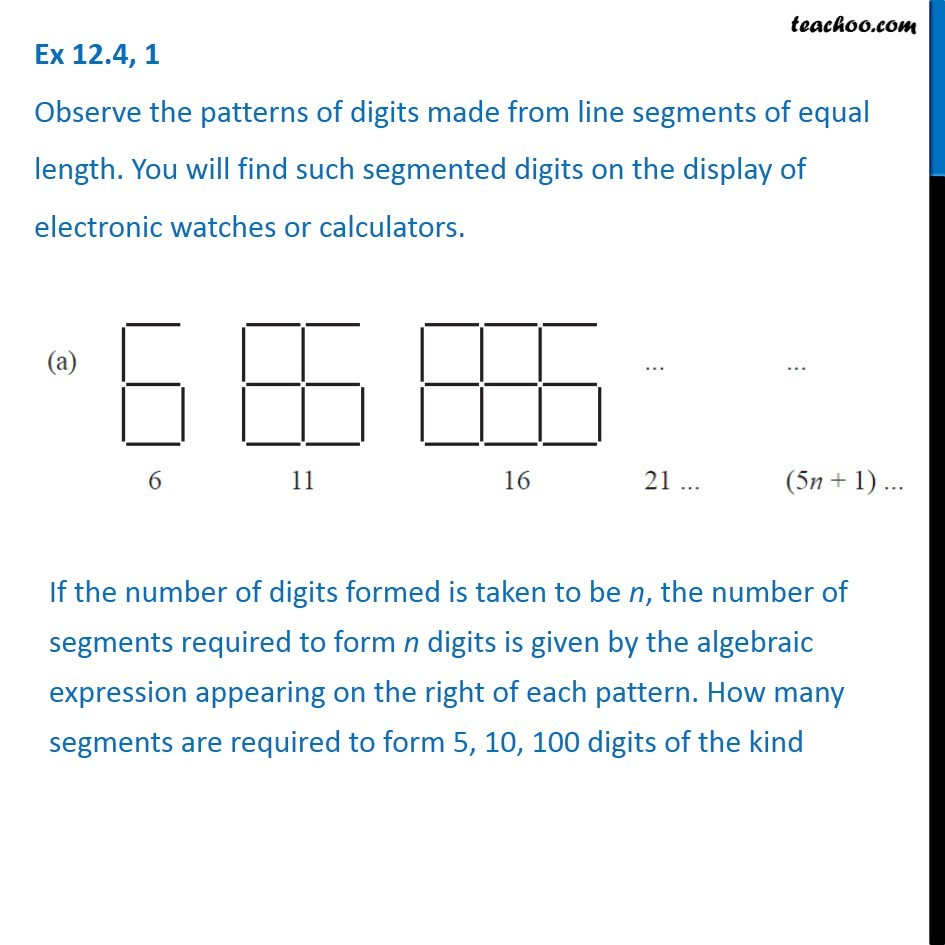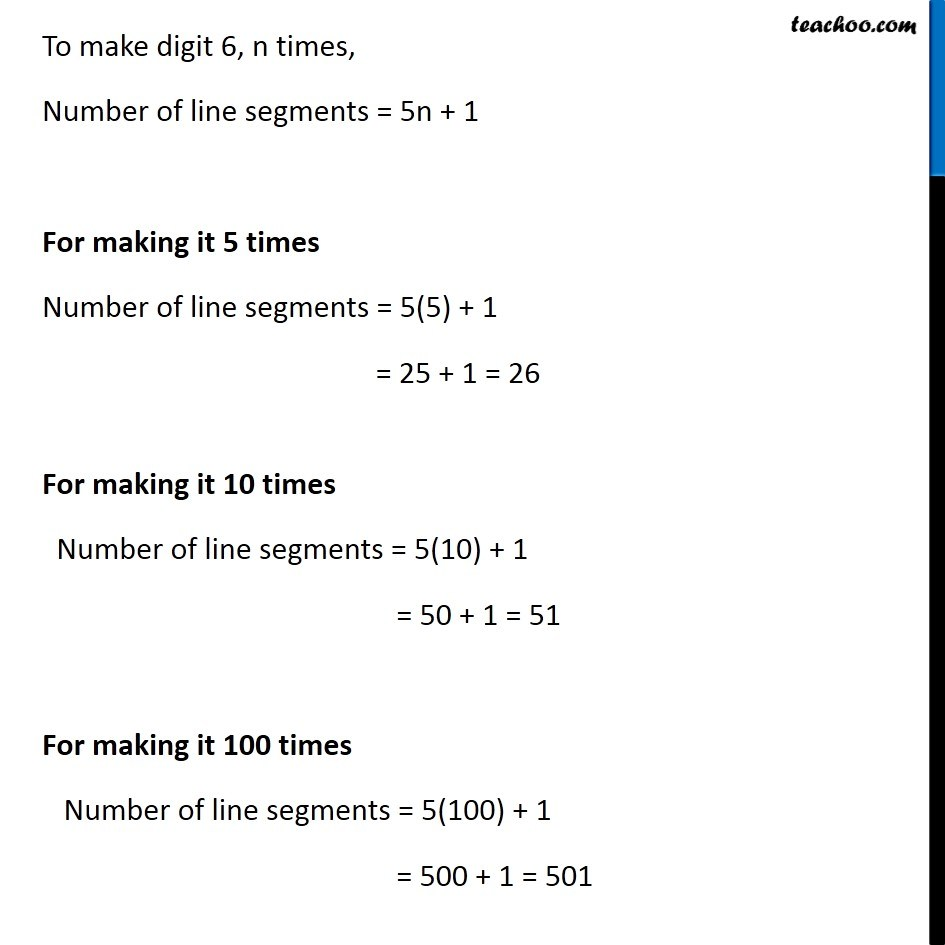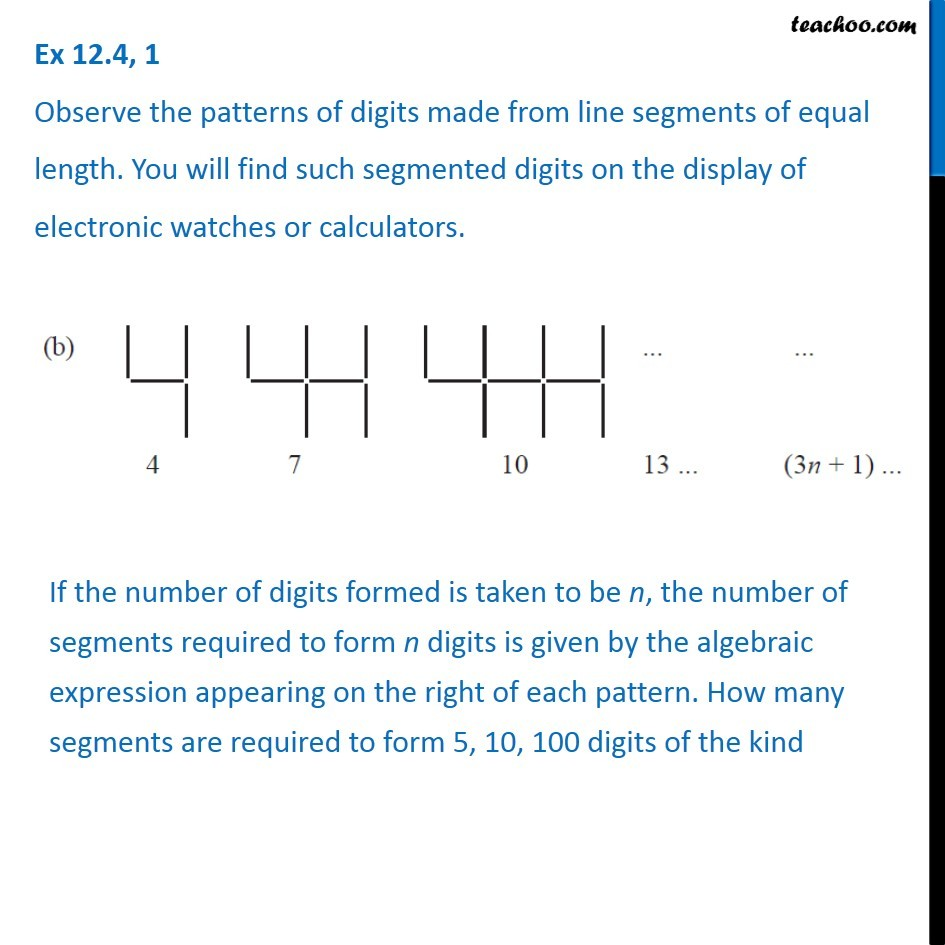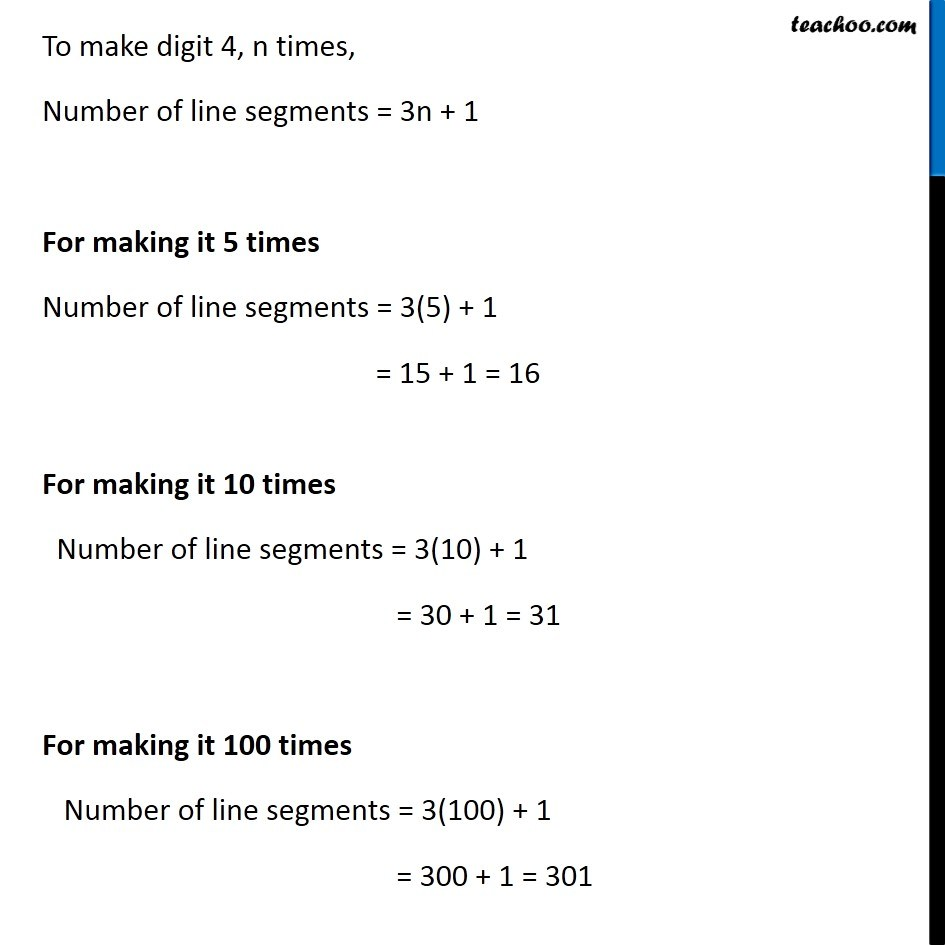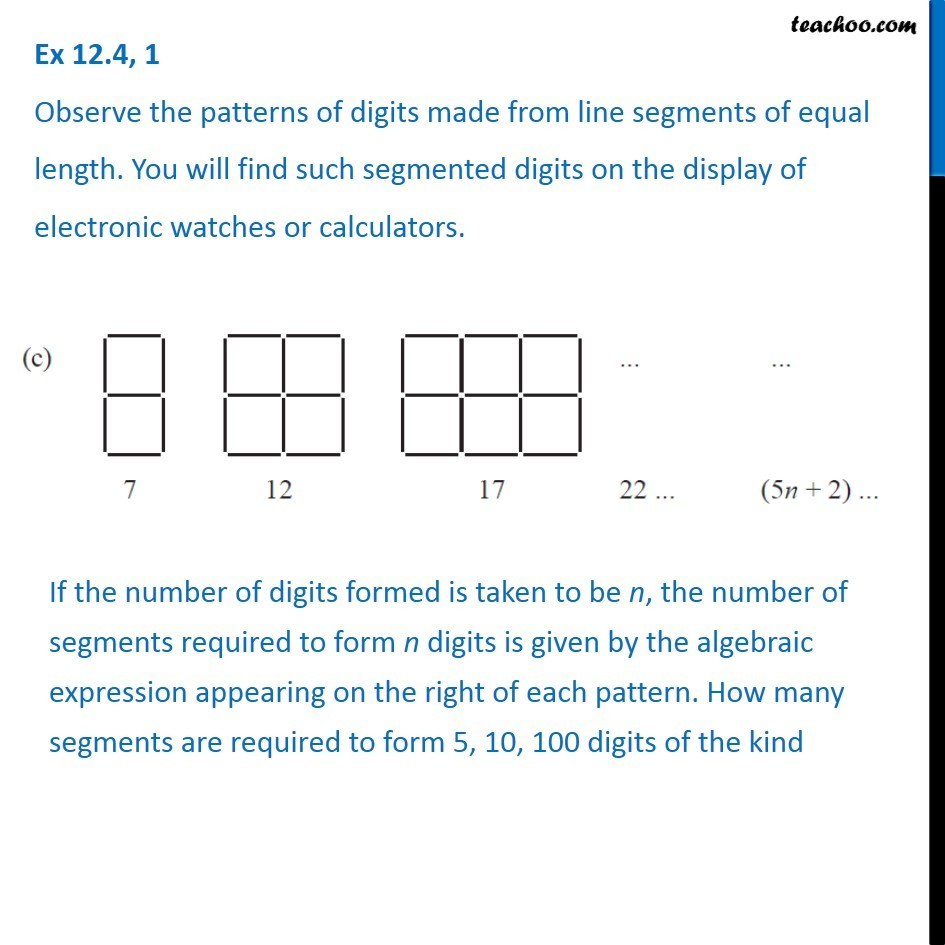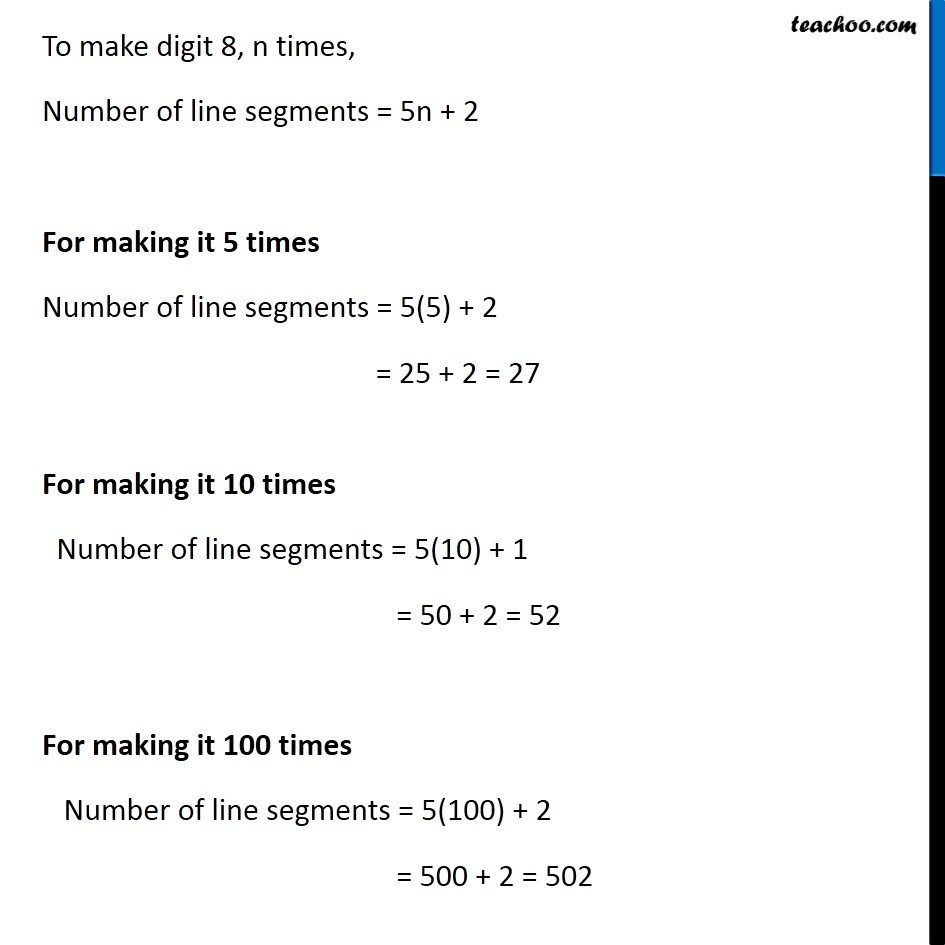### Transcript

Ex 12.4, 1 Observe the patterns of digits made from line segments of equal length. You will find such segmented digits on the display of electronic watches or calculators. If the number of digits formed is taken to be n, the number of segments required to form n digits is given by the algebraic expression appearing on the right of each pattern. How many segments are required to form 5, 10, 100 digits of the kind To make digit 6, n times, Number of line segments = 5n + 1 For making it 5 times Number of line segments = 5(5) + 1 = 25 + 1 = 26 For making it 10 times Number of line segments = 5(10) + 1 = 50 + 1 = 51 For making it 100 times Number of line segments = 5(100) + 1 = 500 + 1 = 501 Ex 12.4, 1 Observe the patterns of digits made from line segments of equal length. You will find such segmented digits on the display of electronic watches or calculators. If the number of digits formed is taken to be n, the number of segments required to form n digits is given by the algebraic expression appearing on the right of each pattern. How many segments are required to form 5, 10, 100 digits of the kind To make digit 4, n times, Number of line segments = 3n + 1 For making it 5 times Number of line segments = 3(5) + 1 = 15 + 1 = 16 For making it 10 times Number of line segments = 3(10) + 1 = 30 + 1 = 31 For making it 100 times Number of line segments = 3(100) + 1 = 300 + 1 = 301 Ex 12.4, 1 Observe the patterns of digits made from line segments of equal length. You will find such segmented digits on the display of electronic watches or calculators. If the number of digits formed is taken to be n, the number of segments required to form n digits is given by the algebraic expression appearing on the right of each pattern. How many segments are required to form 5, 10, 100 digits of the kind To make digit 8, n times, Number of line segments = 5n + 2 For making it 5 times Number of line segments = 5(5) + 2 = 25 + 2 = 27 For making it 10 times Number of line segments = 5(10) + 1 = 50 + 2 = 52 For making it 100 times Number of line segments = 5(100) + 2 = 500 + 2 = 502